• Lectures
• Code
• Notebooks
• Community

# Dynamics in One Dimension¶

## Overview¶

In this lecture we give a quick introduction to discrete time dynamics in one dimension.

In one-dimensional models, the state of the system is described by a single variable.

Although most interesting dynamic models have two or more state variables, the one-dimensional setting is a good place to learn the foundations of dynamics and build intuition.

In :
import numpy as np
import matplotlib.pyplot as plt
%matplotlib inline


## Some Definitions¶

This section sets out the objects of interest and the kinds of properties we study.

### Difference Equations¶

A time homgeneous first order difference equation is an equation of the form

$$x_{t+1} = g(x_t) \tag{1}$$

where $g$ is a function from some subset $S$ of $\mathbb R$ to itself.

Here $S$ is called the state space and $x$ is called the state variable.

In the definition,

• time homogeneity means that $g$ is the same at each time $t$
• first order means dependence on only one lag (i.e., earlier states such as $x_{t-1}$ do not enter into (1)).

If $x_0 \in S$ is given, then (1) recursively defines the sequence

$$x_0, \quad x_1 = g(x_0), \quad x_2 = g(x_1) = g(g(x_0)), \quad \text{etc.} \tag{2}$$

This sequence is called the trajectory of $x_0$ under $g$.

If we define $g^n$ to be $n$ compositions of $g$ with itself, then we can write the trajectory more simply as $x_t = g^t(x_0)$ for $t \geq 0$.

### Example: A Linear Model¶

One simple example is the linear difference equation

$$x_{t+1} = a x_t + b, \qquad S = \mathbb R$$

where $a, b$ are fixed constants.

In this case, given $x_0$, the trajectory (2) is

$$x_0, \quad a x_0 + b, \quad a^2 x_0 + a b + b, \quad \text{etc.} \tag{3}$$

Continuing in this way, and using our knowledge of geometric series, we find that, for any $t \geq 0$,

$$x_t = a^t x_0 + b \frac{1 - a^t}{1 - a} \tag{4}$$

This is about all we need to know about the linear model.

We have an exact expression for $x_t$ for all $t$ and hence a full understanding of the dynamics.

Notice in particular that $|a| < 1$, then, by (4), we have

$$x_t \to \frac{b}{1 - a} \text{ as } t \to \infty \tag{5}$$

regardless of $x_0$

This is an example of what is called global stability, a topic we return to below.

### Example: A Nonlinear Model¶

In the linear example above, we obtained an exact analytical expression for $x_t$ in terms of arbitrary $t$ and $x_0$.

This made analysis of dynamics very easy.

When models are nonlinear, however, the situation can be quite different.

For example, recall how we previously studied the law of motion for the Solow growth model, a simplified version of which is

$$k_{t+1} = s z k_t^{\alpha} + (1 - \delta) k_t \tag{6}$$

Here $k$ is capital stock and $s, z, \alpha, \delta$ are positive parameters with $0 < \alpha, \delta < 1$.

If you try to iterate like we did in (3), you will find that the algebra gets messy quickly.

Analyzing the dynamics of this model requires a different method (see below).

### Stability¶

A steady state of the difference equation $x_{t+1} = g(x_t)$ is a point $x^*$ in $S$ such that $x^* = g(x^*)$.

In other words, $x^*$ is a fixed point of the function $g$ in $S$.

For example, for the linear model $x_{t+1} = a x_t + b$, you can use the definition to check that

• $x^* := b/(1-a)$ is a steady state whenever $a \not= 1$.
• if $a = 1$ and $b=0$, then every $x \in \mathbb R$ is a steady state.
• if $a = 1$ and $b \not= 0$, then the linear model has no steady state in $\mathbb R$.

A steady state $x^*$ of $x_{t+1} = g(x_t)$ is called globally stable if, for all $x_0 \in S$,

$$x_t = g^t(x_0) \to x^* \text{ as } t \to \infty$$

For example, in the linear model $x_{t+1} = a x_t + b$ with $a \not= 1$, the steady state $x^*$

• is globally stable if $|a| < 1$ and
• fails to be globally stable otherwise.

This follows directly from (4).

A steady state $x^*$ of $x_{t+1} = g(x_t)$ is called locally stable if there exists an $\epsilon > 0$ such that

$$| x_0 - x^* | < \epsilon \; \implies \; x_t = g^t(x_0) \to x^* \text{ as } t \to \infty$$

Obviously every globally stable steady state is also locally stable.

We will see examples below where the converse is not true.

## Graphical Analysis¶

We saw above that, for nonlinear models, analyzing dynamics is nontrivial.

There is no single way to tackle all nonlinear models.

However, there is one technique for one-dimensional models that provides a great deal of intuition.

This is a graphical approach based on 45 degree diagrams.

Let’s look at an example: the Solow model with dynamics given in (6).

We begin with some plotting code that you can ignore at first reading.

The function of the code is to produce 45 degree diagrams and time series plots.

In :
def subplots(fs):
"Custom subplots with axes throught the origin"
fig, ax = plt.subplots(figsize=fs)

# Set the axes through the origin
for spine in ['left', 'bottom']:
ax.spines[spine].set_position('zero')
ax.spines[spine].set_color('green')
for spine in ['right', 'top']:
ax.spines[spine].set_color('none')

return fig, ax

def plot45(g, xmin, xmax, x0, num_arrows=6, var='x'):

xgrid = np.linspace(xmin, xmax, 200)

fig, ax = subplots((6.5, 6))
ax.set_xlim(xmin, xmax)
ax.set_ylim(xmin, xmax)

hw = (xmax - xmin) * 0.01
hl = 2 * hw

ax.plot(xgrid, g(xgrid), 'b-', lw=2, alpha=0.6, label='g')
ax.plot(xgrid, xgrid, 'k-', lw=1, alpha=0.7, label='45')

x = x0
xticks = [xmin]
xtick_labels = [xmin]

for i in range(num_arrows):
if i == 0:
ax.arrow(x, 0.0, 0.0, g(x), **arrow_args) # x, y, dx, dy
else:
ax.arrow(x, x, 0.0, g(x) - x, **arrow_args)
ax.plot((x, x), (0, x), 'k', ls='dotted')

ax.arrow(x, g(x), g(x) - x, 0, **arrow_args)
xticks.append(x)
xtick_labels.append(r'${}_{}$'.format(var, str(i)))

x = g(x)
xticks.append(x)
xtick_labels.append(r'${}_{}$'.format(var, str(i+1)))
ax.plot((x, x), (0, x), 'k-', ls='dotted')

xticks.append(xmax)
xtick_labels.append(xmax)
ax.set_xticks(xticks)
ax.set_yticks(xticks)
ax.set_xticklabels(xtick_labels)
ax.set_yticklabels(xtick_labels)

bbox = (0., 1.04, 1., .104)
legend_args = {'bbox_to_anchor': bbox, 'loc': 'upper right'}

ax.legend(ncol=2, frameon=False, **legend_args, fontsize=14)
plt.show()

def ts_plot(g, xmin, xmax, x0, ts_length=6, var='x'):
fig, ax = subplots((7, 5.5))
ax.set_ylim(xmin, xmax)
ax.set_xlabel(r'$t$', fontsize=14)
ax.set_ylabel(r'${}_t$'.format(var), fontsize=14)
x = np.empty(ts_length)
x = x0
for t in range(ts_length-1):
x[t+1] = g(x[t])
ax.plot(range(ts_length),
x,
'bo-',
alpha=0.6,
lw=2,
label=r'${}_t$'.format(var))
ax.legend(loc='best', fontsize=14)
ax.set_xticks(range(ts_length))
plt.show()


Let’s create a 45 degree diagram for the Solow model at a fixed set of parameters

In :
A, s, alpha, delta = 2, 0.3, 0.3, 0.4


Here’s the update function corresponding to the model.

In :
def g(k):
return A * s * k**alpha + (1 - delta) * k


Here is the 45 degree plot.

In :
xmin, xmax = 0, 4  # Suitable plotting region.

plot45(g, xmin, xmax, 0, num_arrows=0)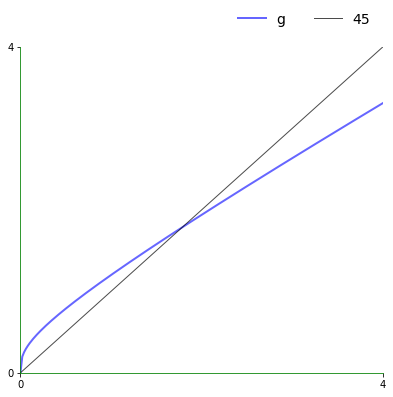The plot shows the function $g$ and the 45 degree line.

Think of $k_t$ as a value on the horizontal axis.

To calculate $k_{t+1}$, we can use the graph of $g$ to see its value on the vertical axis.

Clearly,

• If $g$ lies above the 45 degree line at this point, then we have $k_{t+1} > k_t$.
• If $g$ lies below the 45 degree line at this point, then we have $k_{t+1} < k_t$.
• If $g$ hits the 45 degree line at this point, then we have $k_{t+1} = k_t$, so $k_t$ is a steady state.

For the Solow model, there are two steady states when $S = \mathbb R_+ = [0, \infty)$.

• the origin $k=0$
• the unique positive number such that $k = s z k^{\alpha} + (1 - \delta) k$.

A bit of algebra shows that, in the second case, the steady state is

$$k^* = \left( \frac{sz}{\delta} \right)^{1/(1-\alpha)}$$

### Trajectories¶

By the preceding discussion, in regions where $g$ lies above the 45 degree line, we know that the trajectory is increasing.

The next figure traces out a trajectory in such a region so we can see this more clearly.

The initial condition is $k_0 = 0.25$.

In :
k0 = 0.25

plot45(g, xmin, xmax, k0, num_arrows=5, var='k')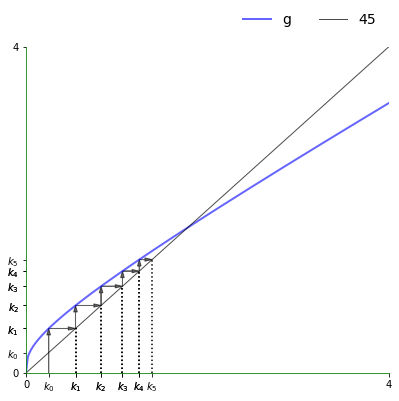We can plot the time series of capital corresponding to the figure above as follows:

In :
ts_plot(g, xmin, xmax, k0, var='k')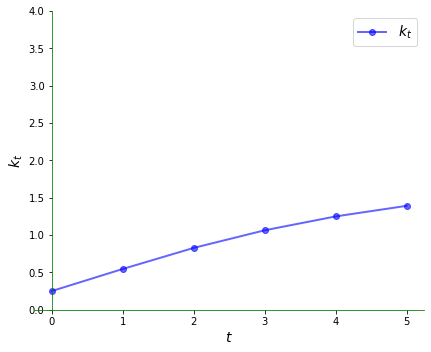Here’s a somewhat longer view:

In :
ts_plot(g, xmin, xmax, k0, ts_length=20, var='k')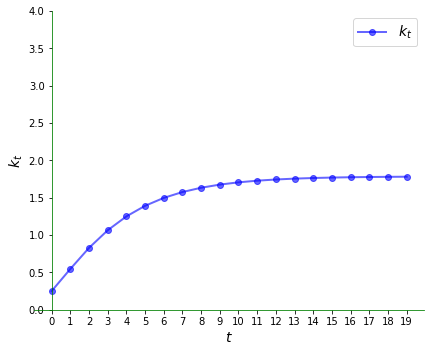When capital stock is higher than the unique positive steady state we see that it declines:

In :
k0 = 2.95

plot45(g, xmin, xmax, k0, num_arrows=5, var='k')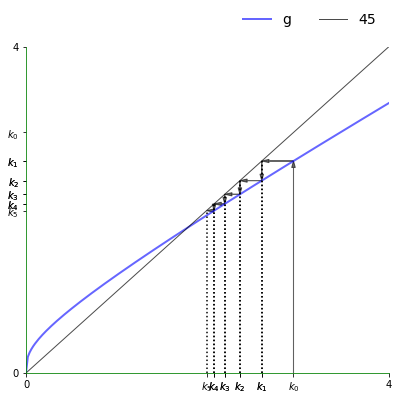Here is the time series:

In :
ts_plot(g, xmin, xmax, k0, var='k')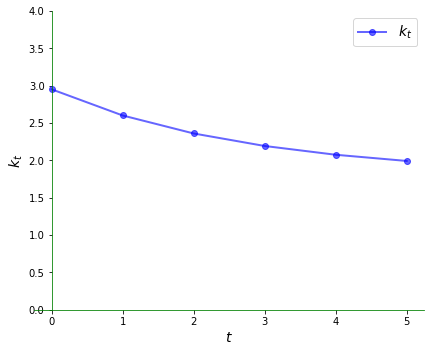### Complex Dynamics¶

The Solow model is nonlinear but still generates very regular dynamics.

One model that generates irregular dynamics is the quadratic map

$$g(x) = 4 x (1 - x), \qquad x \in [0, 1]$$

Let’s have a look at the 45 degree diagram.

In :
xmin, xmax = 0, 1
g = lambda x: 4 * x * (1 - x)

x0 = 0.3
plot45(g, xmin, xmax, x0, num_arrows=0)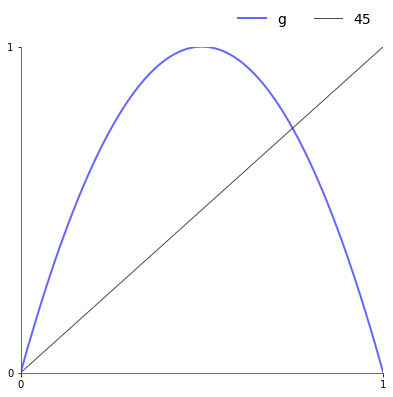Now let’s look at a typical trajectory.

In :
plot45(g, xmin, xmax, x0, num_arrows=6)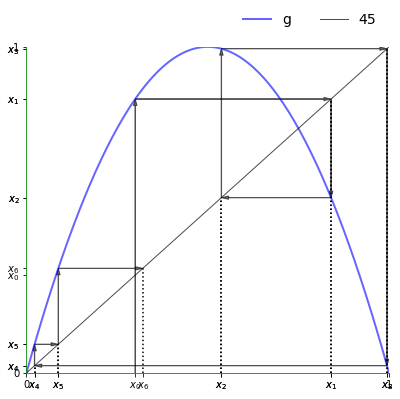Notice how irregular it is.

Here is the corresponding time series plot.

In :
ts_plot(g, xmin, xmax, x0, ts_length=6)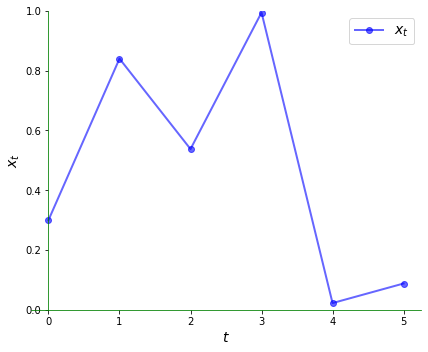The irregularity is even clearer over a longer horizon:

In :
ts_plot(g, xmin, xmax, x0, ts_length=20)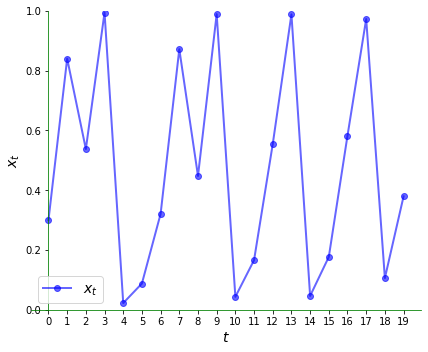## Exercises¶

### Exercise 1¶

Consider again the linear model $x_{t+1} = a x_t + b$ with $a \not=1$.

The unique steady state is $b / (1 - a)$.

The steady state is globally stable if $|a| < 1$.

Try to illustrate this graphically by looking at a range of initial conditions.

What differences do you notice in the cases $a \in (-1, 0)$ and $a \in (0, 1)$?

Use $a=0.5$ and then $a=-0.5$ and study the trajectories

Set $b=1$ throughout.

## Solutions¶

### Exercise 1¶

We will start with the case $a=0.5$.

Let’s set up the model and plotting region:

In :
a, b = 0.5, 1
xmin, xmax = -1, 3
g = lambda x: a * x + b


Now let’s plot a trajectory:

In :
x0 = -0.5
plot45(g, xmin, xmax, x0, num_arrows=5)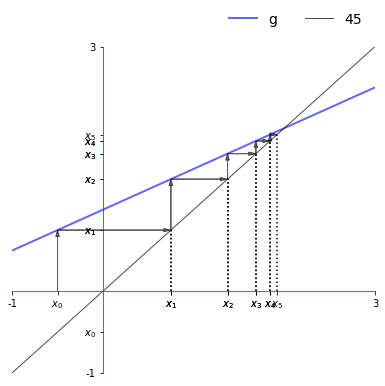Here is the corresponding time series, which converges towards the steady state.

In :
ts_plot(g, xmin, xmax, x0, ts_length=10)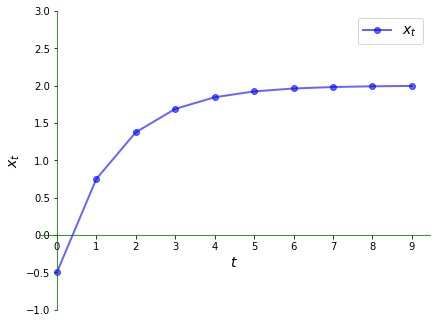Now let’s try $a=-0.5$ and see what differences we observe.

Let’s set up the model and plotting region:

In :
a, b = -0.5, 1
xmin, xmax = -1, 3
g = lambda x: a * x + b


Now let’s plot a trajectory:

In :
x0 = -0.5
plot45(g, xmin, xmax, x0, num_arrows=5)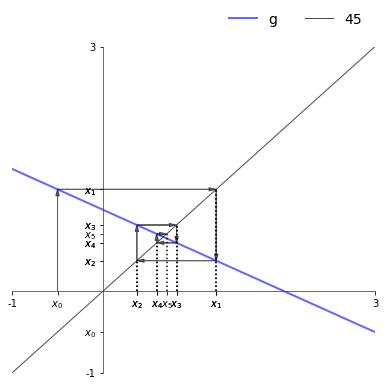Here is the corresponding time series, which converges towards the steady state.

In :
ts_plot(g, xmin, xmax, x0, ts_length=10)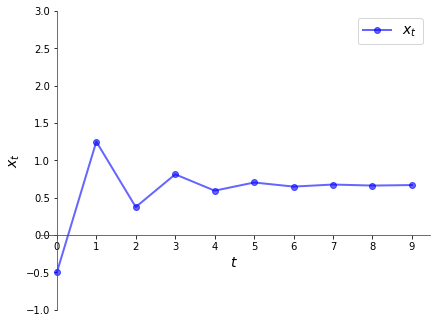Once again we have convergence to the steady state but the nature of convergence differs.

In particular, the time series jumps from above the steady state to below it and back again.

In the current context, the series is said to exhibit damped oscillations.

• Share page